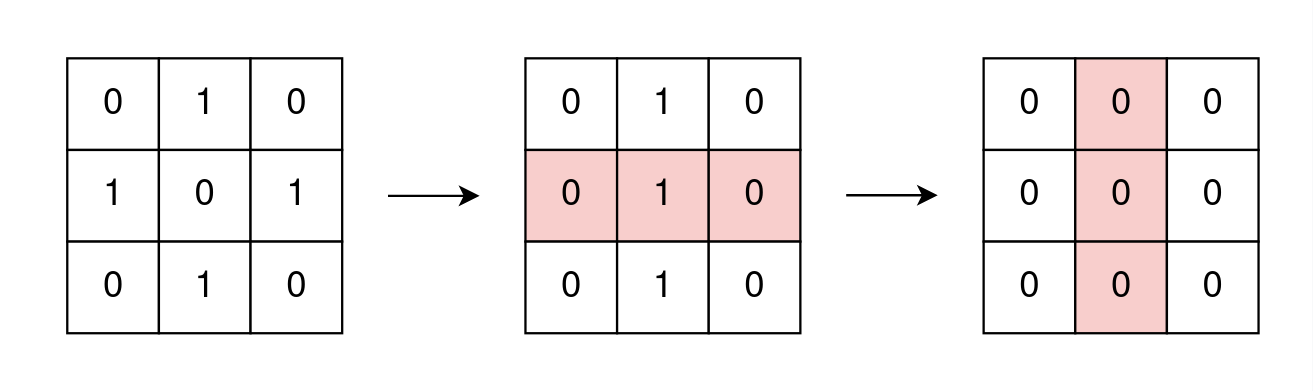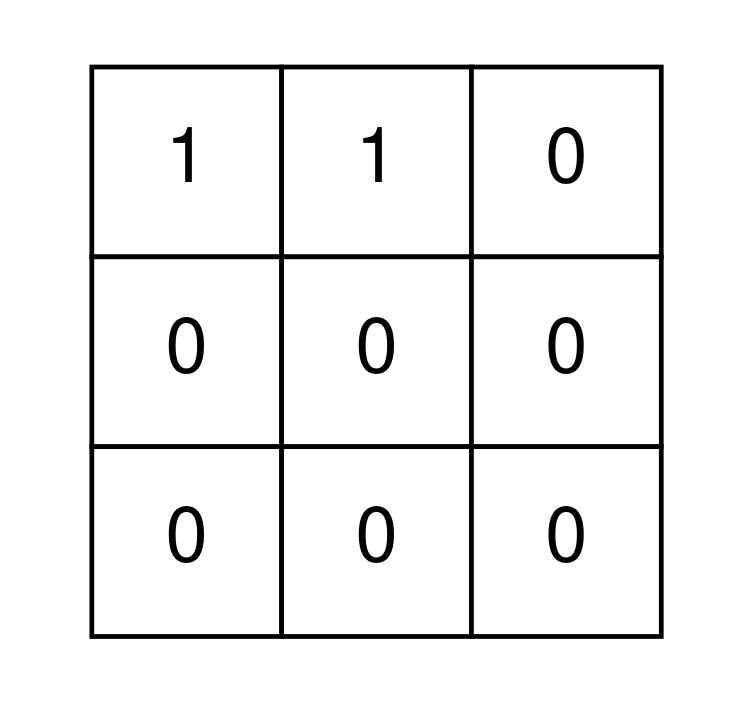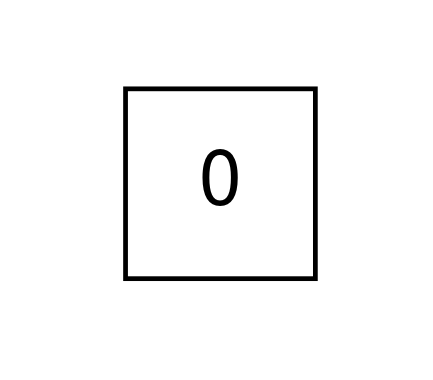##### Welcome to Subscribe On Youtube

Formatted question description: https://leetcode.ca/all/2128.html

# 2128. Remove All Ones With Row and Column Flips (Medium)

You are given an m x n binary matrix grid.

In one operation, you can choose any row or column and flip each value in that row or column (i.e., changing all 0's to 1's, and all 1's to 0's).

Return true if it is possible to remove all 1's from grid using any number of operations or false otherwise.

Example 1:Input: grid = [[0,1,0],[1,0,1],[0,1,0]]
Output: true
Explanation: One possible way to remove all 1's from grid is to:
- Flip the middle row
- Flip the middle column


Example 2:Input: grid = [[1,1,0],[0,0,0],[0,0,0]]
Output: false
Explanation: It is impossible to remove all 1's from grid.


Example 3:Input: grid = []
Output: true
Explanation: There are no 1's in grid.


Constraints:

• m == grid.length
• n == grid[i].length
• 1 <= m, n <= 300
• grid[i][j] is either 0 or 1.

Companies:

Related Topics:
Array, Math, Bit Manipulation, Matrix

Similar Questions:

## Solution

• class Solution {
public boolean removeOnes(int[][] grid) {
Set<String> s = new HashSet<>();
int n = grid.length;
for (var row : grid) {
var cs = new char[n];
for (int i = 0; i < n; ++i) {
cs[i] = (char) (row ^ row[i]);
}
}
return s.size() == 1;
}
}

• class Solution {
public:
bool removeOnes(vector<vector<int>>& grid) {
unordered_set<string> s;
for (auto& row : grid) {
string t;
for (int x : row) {
t.push_back('0' + (row == 0 ? x : x ^ 1));
}
s.insert(t);
}
return s.size() == 1;
}
};

• class Solution:
def removeOnes(self, grid: List[List[int]]) -> bool:
s = set()
for row in grid:
t = tuple(row) if row == grid else tuple(x ^ 1 for x in row)
return len(s) == 1


• func removeOnes(grid [][]int) bool {
s := map[string]bool{}
for _, row := range grid {
t := []byte{}
for _, x := range row {
if row == 1 {
x ^= 1
}
t = append(t, byte(x)+'0')
}
s[string(t)] = true
}
return len(s) == 1
}

• function removeOnes(grid: number[][]): boolean {
const s = new Set<string>();
for (const row of grid) {
if (row === 1) {
for (let i = 0; i < row.length; i++) {
row[i] ^= 1;
}
}
const t = row.join('');